Latest Banking jobs   »   Quantitative Aptitude Quiz For SBI/IBPS PO...

# Quantitative Aptitude Quiz For SBI/IBPS PO Prelims 2021- 15th November

Directions (1-5): In each of these questions, two equations are given. You have to solve both the equations and give answer
(a) if x>y
(b) if x≥y
(c) if x = y or no relation can be established between x and y.
(d) if y>x
(e) if y≥x

Q1. (i) x² – 12x + 32 = 0
(ii) y² – 20y + 96 = 0

Q2. (i) 2x² – 3x – 20 = 0
(ii) 2y² + 11y + 15 = 0

Q3. (i) x² – x – 6 = 0
(ii) y² – 6y + 8 = 0

Q4. (i) x² + 14x – 32 = 0
(ii) y² – y – 12 = 0

Q5. (i) x² – 9x + 20 = 0
(ii) 2y² – 12y + 18 = 0

Directions (6-10): In each of these questions, two equations (I) and (II) are given. You have to solve both the equations and give answer
(a) if x>y
(b) if x≥y
(c) if x<y
(d) if x ≤y
(e) if x = y or no relation can be established between x and y.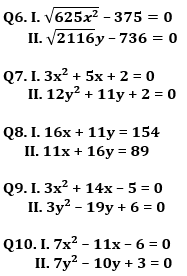Directions (11-15): In the following questions, two equations numbered I and II are given. You have to solve both the equations and give answers among the following options.
(a) if x > y
(b) if x ≥ y
(c) if x < y
(d) if x ≤ y
(e) if x = y or the relationship can’t be established.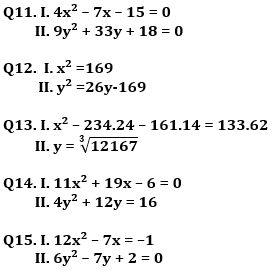Solutions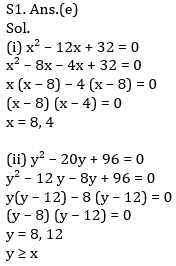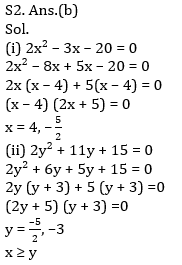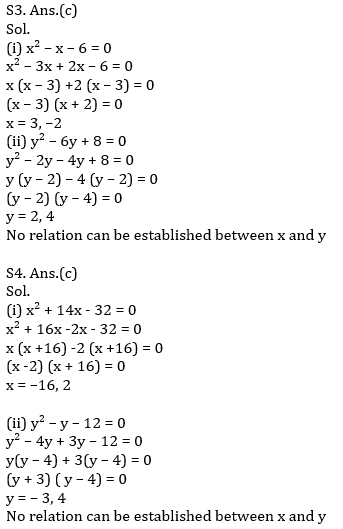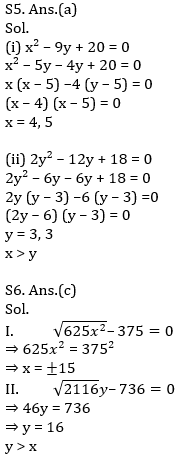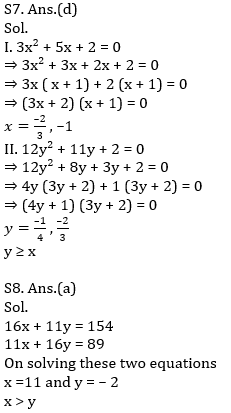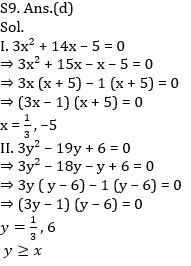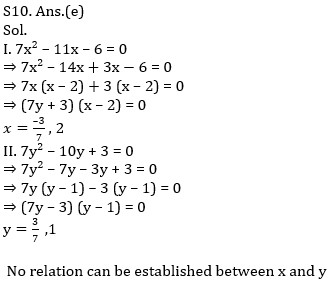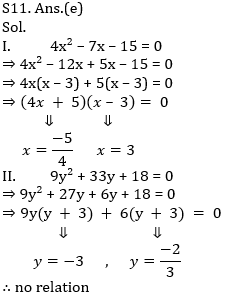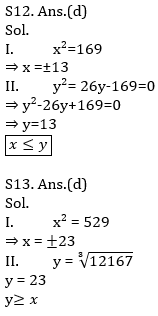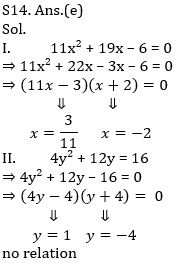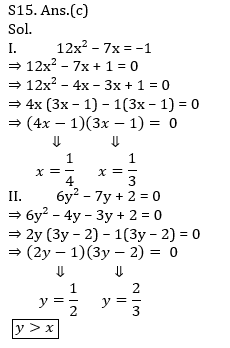×

Thank You, Your details have been submitted we will get back to you.Join India's largest learning destination

What You Will get ?

•Job Alerts
•Daily Quizzes
•Subject-Wise Quizzes
•Current Affairs
•Previous year question papers
•Doubt Solving session

ORJoin India's largest learning destination

What You Will get ?

•Job Alerts
•Daily Quizzes
•Subject-Wise Quizzes
•Current Affairs
•Previous year question papers
•Doubt Solving session

ORJoin India's largest learning destination

What You Will get ?

•Job Alerts
•Daily Quizzes
•Subject-Wise Quizzes
•Current Affairs
•Previous year question papers
•Doubt Solving session

Enter the email address associated with your account, and we'll email you an OTP to verify it's you.Join India's largest learning destination

What You Will get ?

•Job Alerts
•Daily Quizzes
•Subject-Wise Quizzes
•Current Affairs
•Previous year question papers
•Doubt Solving session

Enter OTP

Please enter the OTP sent to
/6

Did not recive OTP?

Resend in 60sJoin India's largest learning destination

What You Will get ?

•Job Alerts
•Daily Quizzes
•Subject-Wise Quizzes
•Current Affairs
•Previous year question papers
•Doubt Solving sessionJoin India's largest learning destination

What You Will get ?

•Job Alerts
•Daily Quizzes
•Subject-Wise Quizzes
•Current Affairs
•Previous year question papers
•Doubt Solving session

Almost there

+91Join India's largest learning destination

What You Will get ?

•Job Alerts
•Daily Quizzes
•Subject-Wise Quizzes
•Current Affairs
•Previous year question papers
•Doubt Solving session

Enter OTP

Please enter the OTP sent to Edit Number

Did not recive OTP?

Resend 60

By skipping this step you will not recieve any free content avalaible on adda247, also you will miss onto notification and job alerts

Are you sure you want to skip this step?

By skipping this step you will not recieve any free content avalaible on adda247, also you will miss onto notification and job alerts

Are you sure you want to skip this step?